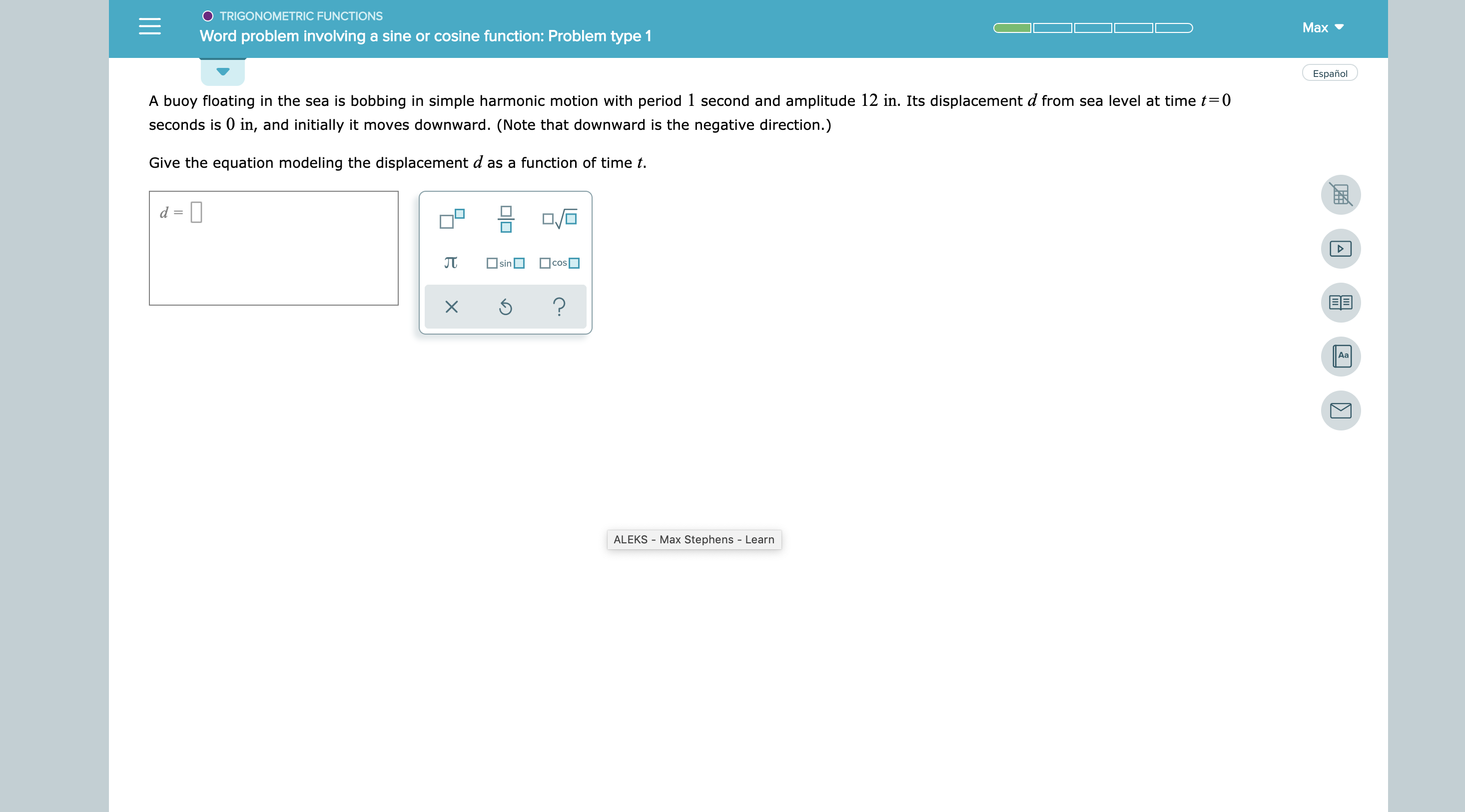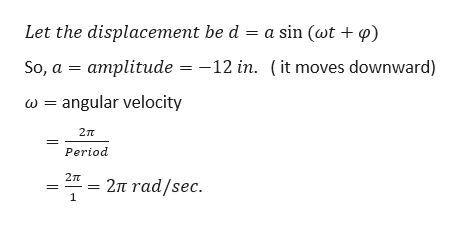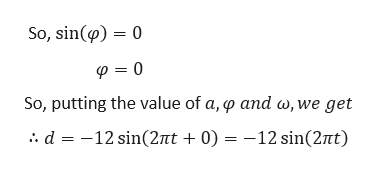# TRIGONOMETRIC FUNCTIONSМаxWord problem involving a sine or cosine function: Problem type 1EspañolA buoy floating in the sea is bobbing in simple harmonic motion with period 1 second and amplitude 12 in. Its displacement d from sea level at time t0seconds is 0 in, and initially it moves downward. (Note that downward is the negative direction.)Give the equation modeling the displacement d as a function of time tcossin?AaALEKS Max Stephens - LearnX

Question
222 views

See attachmenthelp_outlineImage TranscriptioncloseTRIGONOMETRIC FUNCTIONS Маx Word problem involving a sine or cosine function: Problem type 1 Español A buoy floating in the sea is bobbing in simple harmonic motion with period 1 second and amplitude 12 in. Its displacement d from sea level at time t0 seconds is 0 in, and initially it moves downward. (Note that downward is the negative direction.) Give the equation modeling the displacement d as a function of time t cos sin ? Aa ALEKS Max Stephens - Learn X fullscreen
check_circle

Step 1

Given,

Amplitude = 12 in.

Period = 1 seconds.

Since, d at t = 0 is 0 in.help_outlineImage TranscriptioncloseLet the displacement be d a sin (wt + ) So, a amplitude = -12 in. (it moves downward) angular velocity 2T Period 2m 2π Tad/sec . 1 fullscreen
Step 2

Now, at t = 0,...help_outlineImage TranscriptioncloseSo, sin(p) 0 So, putting the value of a, p and w,we get . d 12 sin(2nt 0) 12 sin(27nt) fullscreen

### Want to see the full answer?

See Solution

#### Want to see this answer and more?

Solutions are written by subject experts who are available 24/7. Questions are typically answered within 1 hour.*

See Solution
*Response times may vary by subject and question.
Tagged in

### Other